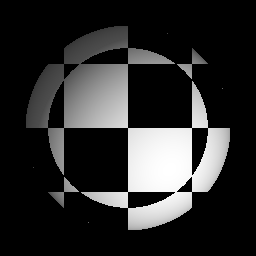# Pattern node¶

The Pattern node outputs a 3D pattern texture generated from common waveform shapes.## Inputs¶

The Pattern node does not accept any input.

## Outputs¶

The Pattern node provides a greyscale 3D texture obtained by mixing a pattern on each X, Y and Z axes.

Many different patterns can be created using this node, do not hesitate to experiment.

## Parameters¶

The Pattern node accepts the following parameters:

• the X, Y and Z parameters define the pattern and their number of repetitions that will be propagated along the X, Y and Y axes. The following patterns are available:

• Sine: the value follows a sinus waveform

• Triangle: the value follows a triangle waveform (changes linearly from 0 to 1 and from 1 to 0)

• Square: the value follows a square waveform (stays constant at 0, then 1)

• Sawtooth: the value follows a sawtooth waveform (changes linearly from 0 to 1, then jumps back to 0)

• Constant: the value is 1

• Bounce: the value follows a half-circle waveform

• the Combiner parameter is the function used to combine the X, Y and Z patterns. The available functions are multiply, add, max, min, xor and pow

## Example images¶